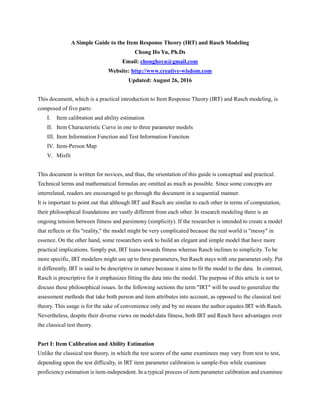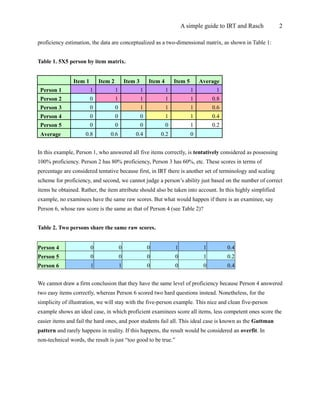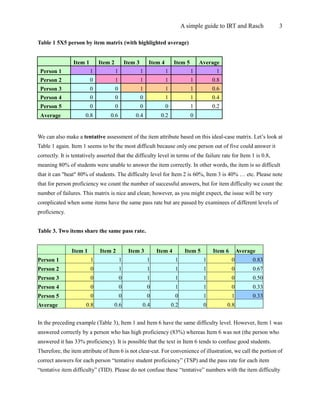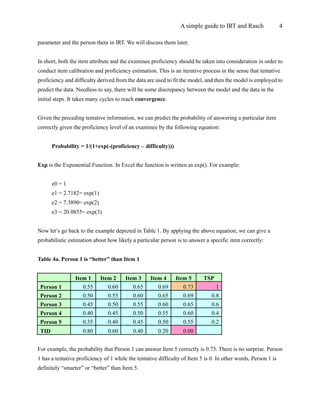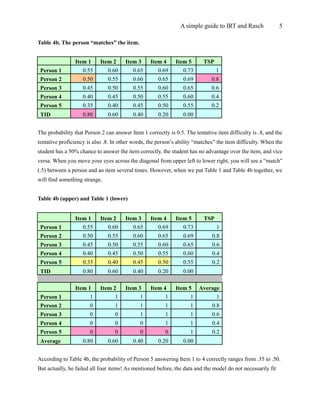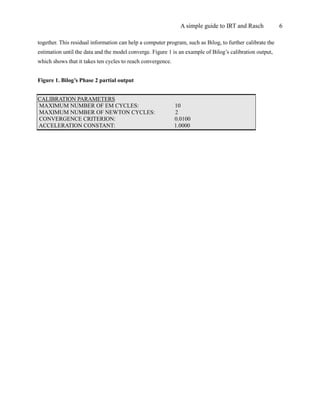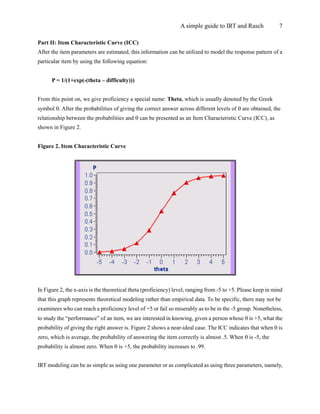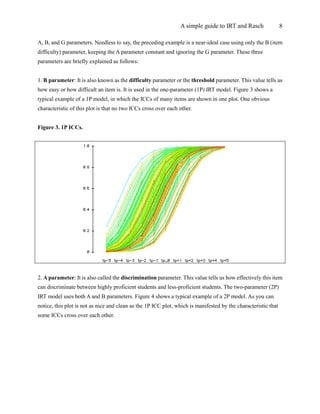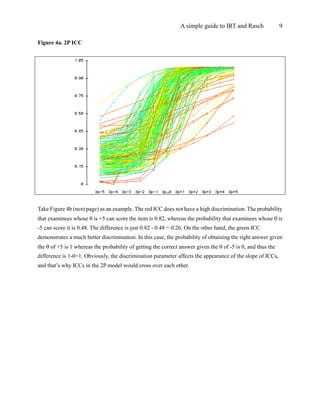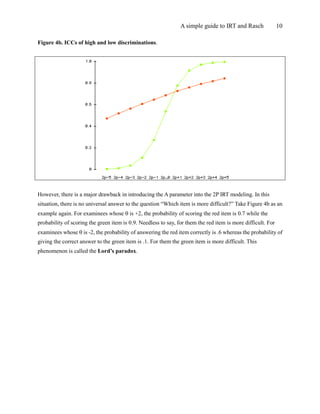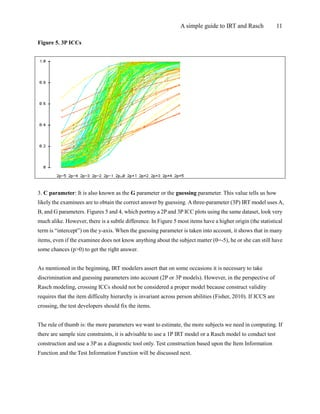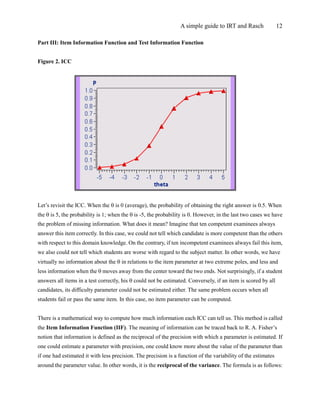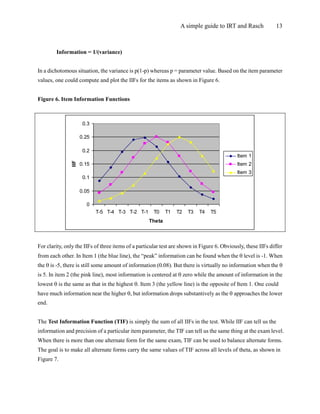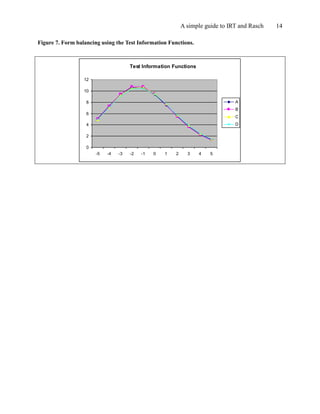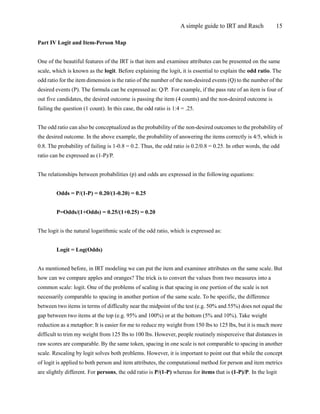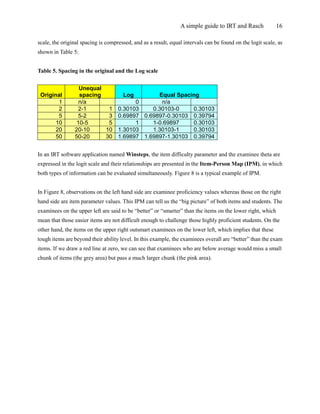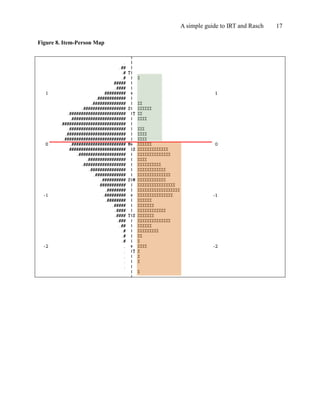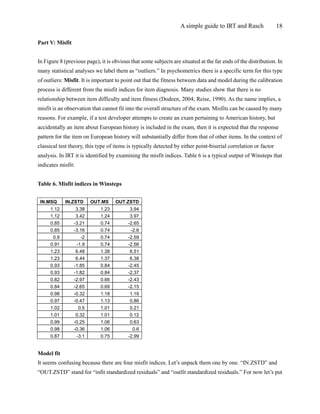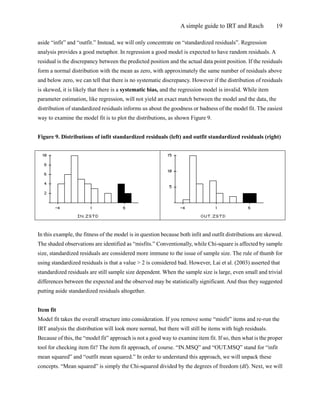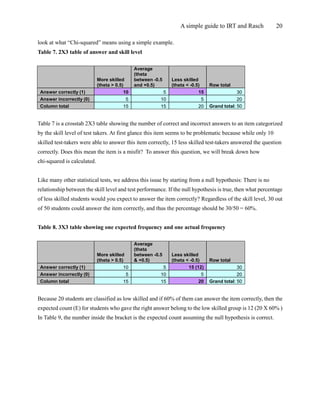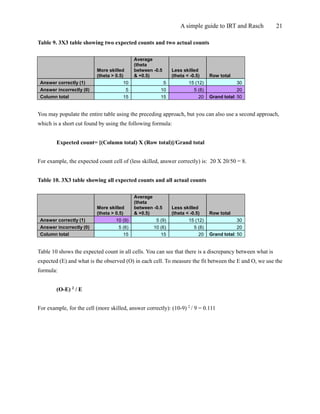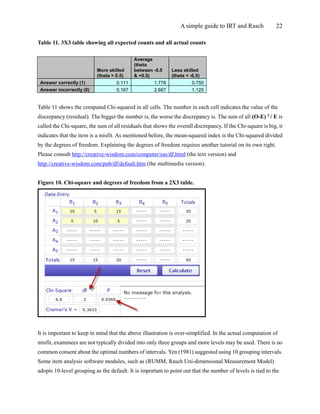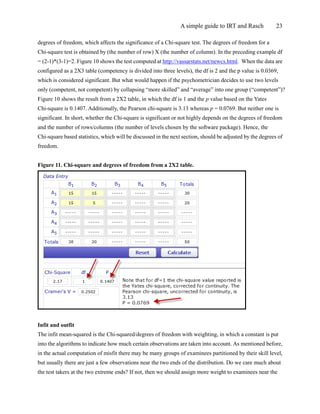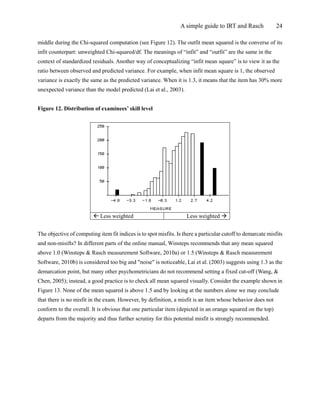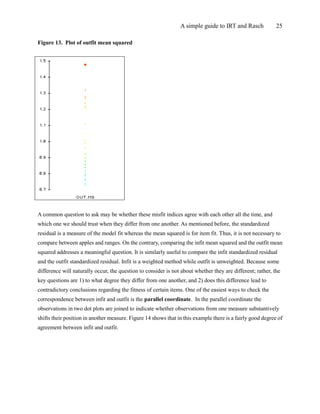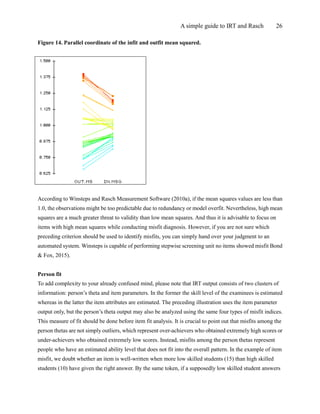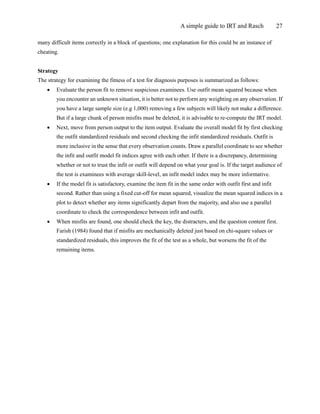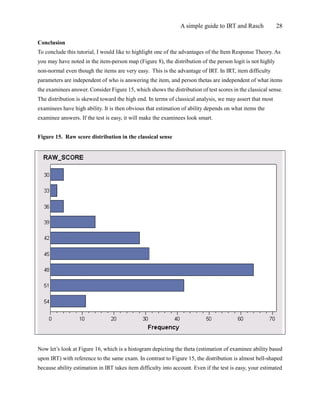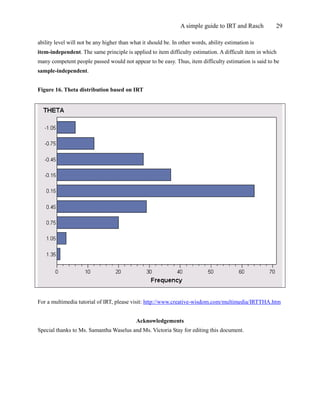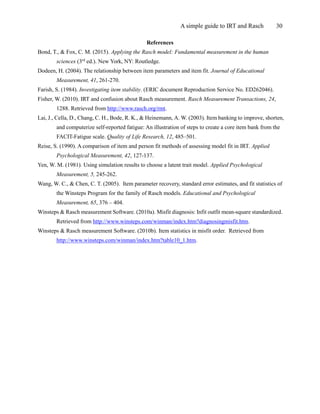Upcoming SlideShare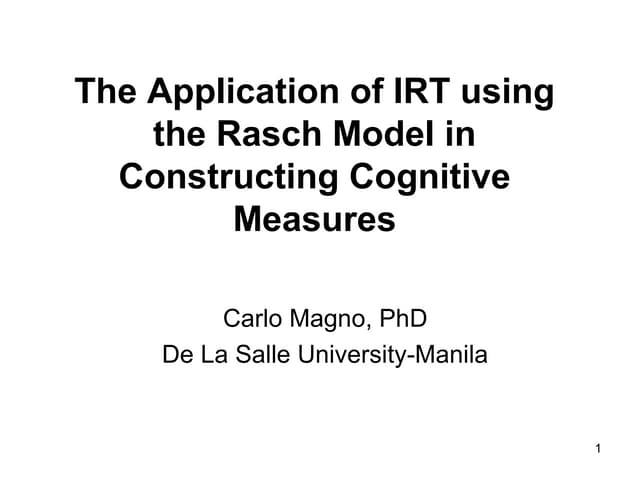The application of irt using the rasch model presnetation1
1 of 30

### A Simple Guide to the Item Response Theory (IRT) and Rasch Modeling

1. A Simple Guide to the Item Response Theory (IRT) and Rasch Modeling Chong Ho Yu, Ph.Ds Email: chonghoyu@gmail.com Website: http://www.creative-wisdom.com Updated: August 26, 2016 This document, which is a practical introduction to Item Response Theory (IRT) and Rasch modeling, is composed of five parts: I. Item calibration and ability estimation II. Item Characteristic Curve in one to three parameter models III. Item Information Function and Test Information Function IV. Item-Person Map V. Misfit This document is written for novices, and thus, the orientation of this guide is conceptual and practical. Technical terms and mathematical formulas are omitted as much as possible. Since some concepts are interrelated, readers are encouraged to go through the document in a sequential manner. It is important to point out that although IRT and Rasch are similar to each other in terms of computation, their philosophical foundations are vastly different from each other. In research modeling there is an ongoing tension between fitness and parsimony (simplicity). If the researcher is intended to create a model that reflects or fits "reality," the model might be very complicated because the real world is "messy" in essence. On the other hand, some researchers seek to build an elegant and simple model that have more practical implications. Simply put, IRT leans towards fitness whereas Rasch inclines to simplicity. To be more specific, IRT modelers might use up to three parameters, but Rasch stays with one parameter only. Put it differently, IRT is said to be descriptive in nature because it aims to fit the model to the data. In contrast, Rasch is prescriptive for it emphasizes fitting the data into the model. The purpose of this article is not to discuss these philosophical issues. In the following sections the term "IRT" will be used to generalize the assessment methods that take both person and item attributes into account, as opposed to the classical test theory. This usage is for the sake of convenience only and by no means the author equates IRT with Rasch. Nevertheless, despite their diverse views on model-data fitness, both IRT and Rasch have advantages over the classical test theory. Part I: Item Calibration and Ability Estimation Unlike the classical test theory, in which the test scores of the same examinees may vary from test to test, depending upon the test difficulty, in IRT item parameter calibration is sample-free while examinee proficiency estimation is item-independent. In a typical process of item parameter calibration and examinee
2. A simple guide to IRT and Rasch 2 proficiency estimation, the data are conceptualized as a two-dimensional matrix, as shown in Table 1: Table 1. 5X5 person by item matrix. Item 1 Item 2 Item 3 Item 4 Item 5 Average Person 1 1 1 1 1 1 1 Person 2 0 1 1 1 1 0.8 Person 3 0 0 1 1 1 0.6 Person 4 0 0 0 1 1 0.4 Person 5 0 0 0 0 1 0.2 Average 0.8 0.6 0.4 0.2 0 In this example, Person 1, who answered all five items correctly, is tentatively considered as possessing 100% proficiency. Person 2 has 80% proficiency, Person 3 has 60%, etc. These scores in terms of percentage are considered tentative because first, in IRT there is another set of terminology and scaling scheme for proficiency, and second, we cannot judge a person’s ability just based on the number of correct items he obtained. Rather, the item attribute should also be taken into account. In this highly simplified example, no examinees have the same raw scores. But what would happen if there is an examinee, say Person 6, whose raw score is the same as that of Person 4 (see Table 2)? Table 2. Two persons share the same raw scores. Person 4 0 0 0 1 1 0.4 Person 5 0 0 0 0 1 0.2 Person 6 1 1 0 0 0 0.4 We cannot draw a firm conclusion that they have the same level of proficiency because Person 4 answered two easy items correctly, whereas Person 6 scored two hard questions instead. Nonetheless, for the simplicity of illustration, we will stay with the five-person example. This nice and clean five-person example shows an ideal case, in which proficient examinees score all items, less competent ones score the easier items and fail the hard ones, and poor students fail all. This ideal case is known as the Guttman pattern and rarely happens in reality. If this happens, the result would be considered an overfit. In non-technical words, the result is just “too good to be true.”
3. A simple guide to IRT and Rasch 3 Table 1 5X5 person by item matrix (with highlighted average) We can also make a tentative assessment of the item attribute based on this ideal-case matrix. Let’s look at Table 1 again. Item 1 seems to be the most difficult because only one person out of five could answer it correctly. It is tentatively asserted that the difficulty level in terms of the failure rate for Item 1 is 0.8, meaning 80% of students were unable to answer the item correctly. In other words, the item is so difficult that it can "beat" 80% of students. The difficulty level for Item 2 is 60%, Item 3 is 40% … etc. Please note that for person proficiency we count the number of successful answers, but for item difficulty we count the number of failures. This matrix is nice and clean; however, as you might expect, the issue will be very complicated when some items have the same pass rate but are passed by examinees of different levels of proficiency. Table 3. Two items share the same pass rate. Item 1 Item 2 Item 3 Item 4 Item 5 Item 6 Average Person 1 1 1 1 1 1 0 0.83 Person 2 0 1 1 1 1 0 0.67 Person 3 0 0 1 1 1 0 0.50 Person 4 0 0 0 1 1 0 0.33 Person 5 0 0 0 0 1 1 0.33 Average 0.8 0.6 0.4 0.2 0 0.8 In the preceding example (Table 3), Item 1 and Item 6 have the same difficulty level. However, Item 1 was answered correctly by a person who has high proficiency (83%) whereas Item 6 was not (the person who answered it has 33% proficiency). It is possible that the text in Item 6 tends to confuse good students. Therefore, the item attribute of Item 6 is not clear-cut. For convenience of illustration, we call the portion of correct answers for each person “tentative student proficiency” (TSP) and the pass rate for each item “tentative item difficulty” (TID). Please do not confuse these “tentative” numbers with the item difficulty Item 1 Item 2 Item 3 Item 4 Item 5 Average Person 1 1 1 1 1 1 1 Person 2 0 1 1 1 1 0.8 Person 3 0 0 1 1 1 0.6 Person 4 0 0 0 1 1 0.4 Person 5 0 0 0 0 1 0.2 Average 0.8 0.6 0.4 0.2 0
4. A simple guide to IRT and Rasch 4 parameter and the person theta in IRT. We will discuss them later. In short, both the item attribute and the examinee proficiency should be taken into consideration in order to conduct item calibration and proficiency estimation. This is an iterative process in the sense that tentative proficiency and difficulty derived from the data are used to fit the model, and then the model is employed to predict the data. Needless to say, there will be some discrepancy between the model and the data in the initial steps. It takes many cycles to reach convergence. Given the preceding tentative information, we can predict the probability of answering a particular item correctly given the proficiency level of an examinee by the following equation: Probability = 1/(1+exp(-(proficiency – difficulty))) Exp is the Exponential Function. In Excel the function is written as exp(). For example: e0 = 1 e1 = 2.7182= exp(1) e2 = 7.3890= exp(2) e3 = 20.0855= exp(3) Now let’s go back to the example depicted in Table 1. By applying the above equation, we can give a probabilistic estimation about how likely a particular person is to answer a specific item correctly: Table 4a. Person 1 is “better” than Item 1 Item 1 Item 2 Item 3 Item 4 Item 5 TSP Person 1 0.55 0.60 0.65 0.69 0.73 1 Person 2 0.50 0.55 0.60 0.65 0.69 0.8 Person 3 0.45 0.50 0.55 0.60 0.65 0.6 Person 4 0.40 0.45 0.50 0.55 0.60 0.4 Person 5 0.35 0.40 0.45 0.50 0.55 0.2 TID 0.80 0.60 0.40 0.20 0.00 For example, the probability that Person 1 can answer Item 5 correctly is 0.73. There is no surprise. Person 1 has a tentative proficiency of 1 while the tentative difficulty of Item 5 is 0. In other words, Person 1 is definitely “smarter” or “better” than Item 5.
5. A simple guide to IRT and Rasch 5 Table 4b. The person “matches” the item. Item 1 Item 2 Item 3 Item 4 Item 5 TSP Person 1 0.55 0.60 0.65 0.69 0.73 1 Person 2 0.50 0.55 0.60 0.65 0.69 0.8 Person 3 0.45 0.50 0.55 0.60 0.65 0.6 Person 4 0.40 0.45 0.50 0.55 0.60 0.4 Person 5 0.35 0.40 0.45 0.50 0.55 0.2 TID 0.80 0.60 0.40 0.20 0.00 The probability that Person 2 can answer Item 1 correctly is 0.5. The tentative item difficulty is .8, and the tentative proficiency is also .8. In other words, the person’s ability “matches” the item difficulty. When the student has a 50% chance to answer the item correctly, the student has no advantage over the item, and vice versa. When you move your eyes across the diagonal from upper left to lower right, you will see a “match” (.5) between a person and an item several times. However, when we put Table 1 and Table 4b together, we will find something strange. Table 4b (upper) and Table 1 (lower) Item 1 Item 2 Item 3 Item 4 Item 5 TSP Person 1 0.55 0.60 0.65 0.69 0.73 1 Person 2 0.50 0.55 0.60 0.65 0.69 0.8 Person 3 0.45 0.50 0.55 0.60 0.65 0.6 Person 4 0.40 0.45 0.50 0.55 0.60 0.4 Person 5 0.35 0.40 0.45 0.50 0.55 0.2 TID 0.80 0.60 0.40 0.20 0.00 Item 1 Item 2 Item 3 Item 4 Item 5 Average Person 1 1 1 1 1 1 1 Person 2 0 1 1 1 1 0.8 Person 3 0 0 1 1 1 0.6 Person 4 0 0 0 1 1 0.4 Person 5 0 0 0 0 1 0.2 Average 0.80 0.60 0.40 0.20 0.00 According to Table 4b, the probability of Person 5 answering Item 1 to 4 correctly ranges from .35 to .50. But actually, he failed all four items! As mentioned before, the data and the model do not necessarily fit
6. A simple guide to IRT and Rasch 6 together. This residual information can help a computer program, such as Bilog, to further calibrate the estimation until the data and the model converge. Figure 1 is an example of Bilog’s calibration output, which shows that it takes ten cycles to reach convergence. Figure 1. Bilog’s Phase 2 partial output CALIBRATION PARAMETERS MAXIMUM NUMBER OF EM CYCLES: 10 MAXIMUM NUMBER OF NEWTON CYCLES: 2 CONVERGENCE CRITERION: 0.0100 ACCELERATION CONSTANT: 1.0000
7. A simple guide to IRT and Rasch 7 Part II: Item Characteristic Curve (ICC) After the item parameters are estimated, this information can be utilized to model the response pattern of a particular item by using the following equation: P = 1/(1+exp(-(theta – difficulty))) From this point on, we give proficiency a special name: Theta, which is usually denoted by the Greek symbol . After the probabilities of giving the correct answer across different levels of  are obtained, the relationship between the probabilities and  can be presented as an Item Characteristic Curve (ICC), as shown in Figure 2. Figure 2. Item Characteristic Curve In Figure 2, the x-axis is the theoretical theta (proficiency) level, ranging from -5 to +5. Please keep in mind that this graph represents theoretical modeling rather than empirical data. To be specific, there may not be examinees who can reach a proficiency level of +5 or fail so miserably as to be in the -5 group. Nonetheless, to study the “performance” of an item, we are interested in knowing, given a person whose  is +5, what the probability of giving the right answer is. Figure 2 shows a near-ideal case. The ICC indicates that when  is zero, which is average, the probability of answering the item correctly is almost .5. When  is -5, the probability is almost zero. When  is +5, the probability increases to .99. IRT modeling can be as simple as using one parameter or as complicated as using three parameters, namely,
8. A simple guide to IRT and Rasch 8 A, B, and G parameters. Needless to say, the preceding example is a near-ideal case using only the B (item difficulty) parameter, keeping the A parameter constant and ignoring the G parameter. These three parameters are briefly explained as follows: 1. B parameter: It is also known as the difficulty parameter or the threshold parameter. This value tells us how easy or how difficult an item is. It is used in the one-parameter (1P) IRT model. Figure 3 shows a typical example of a 1P model, in which the ICCs of many items are shown in one plot. One obvious characteristic of this plot is that no two ICCs cross over each other. Figure 3. 1P ICCs. 2. A parameter: It is also called the discrimination parameter. This value tells us how effectively this item can discriminate between highly proficient students and less-proficient students. The two-parameter (2P) IRT model uses both A and B parameters. Figure 4 shows a typical example of a 2P model. As you can notice, this plot is not as nice and clean as the 1P ICC plot, which is manifested by the characteristic that some ICCs cross over each other.
9. A simple guide to IRT and Rasch 9 Figure 4a. 2P ICC Take Figure 4b (next page) as an example. The red ICC does not have a high discrimination. The probability that examinees whose  is +5 can score the item is 0.82, whereas the probability that examinees whose  is -5 can score it is 0.48. The difference is just 0.82 - 0.48 = 0.26. On the other hand, the green ICC demonstrates a much better discrimination. In this case, the probability of obtaining the right answer given the  of +5 is 1 whereas the probability of getting the correct answer given the  of -5 is 0, and thus the difference is 1-0=1. Obviously, the discrimination parameter affects the appearance of the slope of ICCs, and that’s why ICCs in the 2P model would cross over each other.
10. A simple guide to IRT and Rasch 10 Figure 4b. ICCs of high and low discriminations. However, there is a major drawback in introducing the A parameter into the 2P IRT modeling. In this situation, there is no universal answer to the question “Which item is more difficult?” Take Figure 4b as an example again. For examinees whose  is +2, the probability of scoring the red item is 0.7 while the probability of scoring the green item is 0.9. Needless to say, for them the red item is more difficult. For examinees whose  is -2, the probability of answering the red item correctly is .6 whereas the probability of giving the correct answer to the green item is .1. For them the green item is more difficult. This phenomenon is called the Lord’s paradox.
11. A simple guide to IRT and Rasch 11 Figure 5. 3P ICCs 3. C parameter: It is also known as the G parameter or the guessing parameter. This value tells us how likely the examinees are to obtain the correct answer by guessing. Athree-parameter (3P) IRT model uses A, B, and G parameters. Figures 5 and 4, which portray a 2P and 3P ICC plots using the same dataset, look very much alike. However, there is a subtle difference. In Figure 5 most items have a higher origin (the statistical term is “intercept”) on the y-axis. When the guessing parameter is taken into account, it shows that in many items, even if the examinee does not know anything about the subject matter (=-5), he or she can still have some chances (p>0) to get the right answer. As mentioned in the beginning, IRT modelers assert that on some occasions it is necessary to take discrimination and guessing parameters into account (2P or 3P models). However, in the perspective of Rasch modeling, crossing ICCs should not be considered a proper model because construct validity requires that the item difficulty hierarchy is invariant across person abilities (Fisher, 2010). If ICCS are crossing, the test developers should fix the items. The rule of thumb is: the more parameters we want to estimate, the more subjects we need in computing. If there are sample size constraints, it is advisable to use a 1P IRT model or a Rasch model to conduct test construction and use a 3P as a diagnostic tool only. Test construction based upon the Item Information Function and the Test Information Function will be discussed next.
12. A simple guide to IRT and Rasch 12 Part III: Item Information Function and Test Information Function Figure 2. ICC Let’s revisit the ICC. When the  is 0 (average), the probability of obtaining the right answer is 0.5. When the  is 5, the probability is 1; when the  is -5, the probability is 0. However, in the last two cases we have the problem of missing information. What does it mean? Imagine that ten competent examinees always answer this item correctly. In this case, we could not tell which candidate is more competent than the others with respect to this domain knowledge. On the contrary, if ten incompetent examinees always fail this item, we also could not tell which students are worse with regard to the subject matter. In other words, we have virtually no information about the  in relations to the item parameter at two extreme poles, and less and less information when the  moves away from the center toward the two ends. Not surprisingly, if a student answers all items in a test correctly, his  could not be estimated. Conversely, if an item is scored by all candidates, its difficulty parameter could not be estimated either. The same problem occurs when all students fail or pass the same item. In this case, no item parameter can be computed. There is a mathematical way to compute how much information each ICC can tell us. This method is called the Item Information Function (IIF). The meaning of information can be traced back to R. A. Fisher’s notion that information is defined as the reciprocal of the precision with which a parameter is estimated. If one could estimate a parameter with precision, one could know more about the value of the parameter than if one had estimated it with less precision. The precision is a function of the variability of the estimates around the parameter value. In other words, it is the reciprocal of the variance. The formula is as follows:
13. A simple guide to IRT and Rasch 13 Information = 1/(variance) In a dichotomous situation, the variance is p(1-p) whereas p = parameter value. Based on the item parameter values, one could compute and plot the IIFs for the items as shown in Figure 6. Figure 6. Item Information Functions 0 0.05 0.1 0.15 0.2 0.25 0.3 T-5 T-4 T-3 T-2 T-1 T0 T1 T2 T3 T4 T5 Theta IIF Item 1 Item 2 Item 3 For clarity, only the IIFs of three items of a particular test are shown in Figure 6. Obviously, these IIFs differ from each other. In Item 1 (the blue line), the “peak” information can be found when the  level is -1. When the  is -5, there is still some amount of information (0.08). But there is virtually no information when the  is 5. In item 2 (the pink line), most information is centered at  zero while the amount of information in the lowest  is the same as that in the highest . Item 3 (the yellow line) is the opposite of Item 1. One could have much information near the higher , but information drops substantively as the approaches the lower end. The Test Information Function (TIF) is simply the sum of all IIFs in the test. While IIF can tell us the information and precision of a particular item parameter, the TIF can tell us the same thing at the exam level. When there is more than one alternate form for the same exam, TIF can be used to balance alternate forms. The goal is to make all alternate forms carry the same values of TIF across all levels of theta, as shown in Figure 7.
14. A simple guide to IRT and Rasch 14 Figure 7. Form balancing using the Test Information Functions. Test Information Functions 0 2 4 6 8 10 12 -5 -4 -3 -2 -1 0 1 2 3 4 5 A B C D
15. A simple guide to IRT and Rasch 15 Part IV Logit and Item-Person Map One of the beautiful features of the IRT is that item and examinee attributes can be presented on the same scale, which is known as the logit. Before explaining the logit, it is essential to explain the odd ratio. The odd ratio for the item dimension is the ratio of the number of the non-desired events (Q) to the number of the desired events (P). The formula can be expressed as: Q/P. For example, if the pass rate of an item is four of out five candidates, the desired outcome is passing the item (4 counts) and the non-desired outcome is failing the question (1 count). In this case, the odd ratio is 1:4 = .25. The odd ratio can also be conceptualized as the probability of the non-desired outcomes to the probability of the desired outcome. In the above example, the probability of answering the items correctly is 4/5, which is 0.8. The probability of failing is 1-0.8 = 0.2. Thus, the odd ratio is 0.2/0.8 = 0.25. In other words, the odd ratio can be expressed as (1-P)/P. The relationships between probabilities (p) and odds are expressed in the following equations: Odds = P/(1-P) = 0.20/(1-0.20) = 0.25 P=Odds/(1+Odds) = 0.25/(1+0.25) = 0.20 The logit is the natural logarithmic scale of the odd ratio, which is expressed as: Logit = Log(Odds) As mentioned before, in IRT modeling we can put the item and examinee attributes on the same scale. But how can we compare apples and oranges? The trick is to convert the values from two measures into a common scale: logit. One of the problems of scaling is that spacing in one portion of the scale is not necessarily comparable to spacing in another portion of the same scale. To be specific, the difference between two items in terms of difficulty near the midpoint of the test (e.g. 50% and 55%) does not equal the gap between two items at the top (e.g. 95% and 100%) or at the bottom (5% and 10%). Take weight reduction as a metaphor: It is easier for me to reduce my weight from 150 lbs to 125 lbs, but it is much more difficult to trim my weight from 125 lbs to 100 lbs. However, people routinely misperceive that distances in raw scores are comparable. By the same token, spacing in one scale is not comparable to spacing in another scale. Rescaling by logit solves both problems. However, it is important to point out that while the concept of logit is applied to both person and item attributes, the computational method for person and item metrics are slightly different. For persons, the odd ratio is P/(1-P) whereas for items that is (1-P)/P. In the logit
16. A simple guide to IRT and Rasch 16 scale, the original spacing is compressed, and as a result, equal intervals can be found on the logit scale, as shown in Table 5: Table 5. Spacing in the original and the Log scale Original Unequal spacing Log Equal Spacing 1 n/a 0 n/a 2 2-1 1 0.30103 0.30103-0 0.30103 5 5-2 3 0.69897 0.69897-0.30103 0.39794 10 10-5 5 1 1-0.69897 0.30103 20 20-10 10 1.30103 1.30103-1 0.30103 50 50-20 30 1.69897 1.69897-1.30103 0.39794 In an IRT software application named Winsteps, the item difficulty parameter and the examinee theta are expressed in the logit scale and their relationships are presented in the Item-Person Map (IPM), in which both types of information can be evaluated simultaneously. Figure 8 is a typical example of IPM. In Figure 8, observations on the left hand side are examinee proficiency values whereas those on the right hand side are item parameter values. This IPM can tell us the “big picture” of both items and students. The examinees on the upper left are said to be “better” or “smarter” than the items on the lower right, which mean that those easier items are not difficult enough to challenge those highly proficient students. On the other hand, the items on the upper right outsmart examinees on the lower left, which implies that these tough items are beyond their ability level. In this example, the examinees overall are “better” than the exam items. If we draw a red line at zero, we can see that examinees who are below average would miss a small chunk of items (the grey area) but pass a much larger chunk (the pink area).
17. A simple guide to IRT and Rasch 17 Figure 8. Item-Person Map
18. A simple guide to IRT and Rasch 18 Part V: Misfit In Figure 8 (previous page), it is obvious that some subjects are situated at the far ends of the distribution. In many statistical analyses we label them as “outliers.” In psychometrics there is a specific term for this type of outliers: Misfit. It is important to point out that the fitness between data and model during the calibration process is different from the misfit indices for item diagnosis. Many studies show that there is no relationship between item difficulty and item fitness (Dodeen, 2004; Reise, 1990). As the name implies, a misfit is an observation that cannot fit into the overall structure of the exam. Misfits can be caused by many reasons. For example, if a test developer attempts to create an exam pertaining to American history, but accidentally an item about European history is included in the exam, then it is expected that the response pattern for the item on European history will substantially differ from that of other items. In the context of classical test theory, this type of items is typically detected by either point-biserial correlation or factor analysis. In IRT it is identified by examining the misfit indices. Table 6 is a typical output of Winsteps that indicates misfit: Table 6. Misfit indices in Winsteps IN.MSQ IN.ZSTD OUT.MS OUT.ZSTD 1.12 3.38 1.23 3.94 1.12 3.42 1.24 3.97 0.85 -3.21 0.74 -2.65 0.85 -3.16 0.74 -2.6 0.9 -2 0.74 -2.59 0.91 -1.9 0.74 -2.56 1.23 6.48 1.38 6.51 1.23 6.44 1.37 6.38 0.93 -1.85 0.84 -2.45 0.93 -1.82 0.84 -2.37 0.82 -2.97 0.66 -2.43 0.84 -2.65 0.69 -2.15 0.98 -0.32 1.18 1.16 0.97 -0.47 1.13 0.86 1.02 0.5 1.01 0.21 1.01 0.32 1.01 0.12 0.99 -0.25 1.06 0.63 0.98 -0.36 1.06 0.6 0.87 -3.1 0.75 -2.99 Model fit It seems confusing because there are four misfit indices. Let’s unpack them one by one. “IN.ZSTD” and “OUT.ZSTD” stand for “infit standardized residuals” and “outfit standardized residuals.” For now let’s put
19. A simple guide to IRT and Rasch 19 aside “infit” and “outfit.” Instead, we will only concentrate on “standardized residuals”. Regression analysis provides a good metaphor. In regression a good model is expected to have random residuals. A residual is the discrepancy between the predicted position and the actual data point position. If the residuals form a normal distribution with the mean as zero, with approximately the same number of residuals above and below zero, we can tell that there is no systematic discrepancy. However if the distribution of residuals is skewed, it is likely that there is a systematic bias, and the regression model is invalid. While item parameter estimation, like regression, will not yield an exact match between the model and the data, the distribution of standardized residuals informs us about the goodness or badness of the model fit. The easiest way to examine the model fit is to plot the distributions, as shown Figure 9. Figure 9. Distributions of infit standardized residuals (left) and outfit standardized residuals (right) In this example, the fitness of the model is in question because both infit and outfit distributions are skewed. The shaded observations are identified as “misfits.” Conventionally, while Chi-square is affected by sample size, standardized residuals are considered more immune to the issue of sample size. The rule of thumb for using standardized residuals is that a value > 2 is considered bad. However, Lai et al. (2003) asserted that standardized residuals are still sample size dependent. When the sample size is large, even small and trivial differences between the expected and the observed may be statistically significant. And thus they suggested putting aside standardized residuals altogether. Item fit Model fit takes the overall structure into consideration. If you remove some “misfit” items and re-run the IRT analysis the distribution will look more normal, but there will still be items with high residuals. Because of this, the “model fit” approach is not a good way to examine item fit. If so, then what is the proper tool for checking item fit? The item fit approach, of course. “IN.MSQ” and “OUT.MSQ” stand for “infit mean squared” and “outfit mean squared.” In order to understand this approach, we will unpack these concepts. “Mean squared” is simply the Chi-squared divided by the degrees of freedom (df). Next, we will